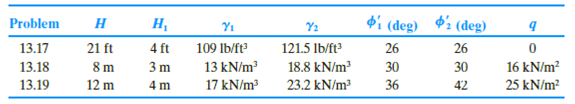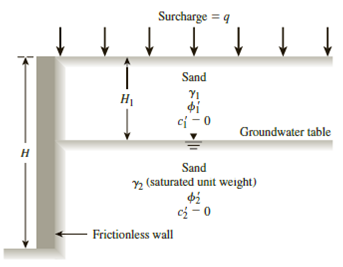Chapter 13, Problem 13.19PPrinciples of Geotechnical Enginee...

9th Edition
Braja M. Das + 1 other
ISBN: 9781305970939

Solutions

Chapter
SectionPrinciples of Geotechnical Enginee...

9th Edition
Braja M. Das + 1 other
ISBN: 9781305970939
Textbook Problem

13.17 through 13.19 A retaining wall is shown in Figure 13.41. For each problem, determine the Rankine active force, Pa, per unit length of the wall and the location of the resultant.Figure 13.41

To determine

Find the Rankine active force Pa per unit length of the wall and the location z¯ of the resultant force.

Explanation

Given information:

The height (H) of the retaining wall is 12 m.

The depth H1 of sand is 4.0 m.

The unit weight γ1 of the sand is 17kN/m3.

The sand friction angle ϕ1 is 36°.

The cohesion c1 of sand is 0.

The surcharge pressure (q) is 25kN/m2.

The depth H2 of saturated sand is 12 m.

The saturated unit weight γ2 of the sand is 23.2kN/m3.

The saturated sand friction angle ϕ2 is 42°.

The cohesion c2 of saturated sand is 0.

Calculation:

For sand:

Determine the active earth pressure coefficient Ka using the formula.

Ka=tan2(45ϕ2)

Substitute 36° for ϕ.

Ka=tan2(4536°2)=tan2(4518)=tan2(27)=0.259

For saturated sand:

Determine the active earth pressure coefficient Ka using the formula.

Ka=tan2(45ϕ2)

Substitute 42° for ϕ.

Ka=tan2(4542°2)=tan2(4521)=tan2(24)=0.198

Determine the total stress σo at 0 m depth using the relation.

σo=q

Substitute 25kN/m2 for q.

σo=25kN/m2

Determine the pore water pressure at 0 m depth using the relation.

u=γw×h

Here, γw is the unit weight of the water.

Take the unit weight of the water as 9.81kN/m3.

Substitute 9.81kN/m3 for γw and 0 m for h.

u=9.81×0=0

Determine the effective active earth pressure σa at 0 m depth using the relation.

σa=σoKa

Substitute 25kN/m2 for σo and 0.259 for Ka.

σa=25(0.259)=6.47kN/m2

Determine the total stress (sand) σo at 4 m depth using the relation.

σo=q+γ1×H1

Substitute 25kN/m2 for q, 17kN/m3 γ1, and 4.0 m for H1.

σo=25+17×4.0=93kN/m2

Determine the total stress (saturated sand) σo at 4 m depth using the relation.

σo=q+γ2×H2

Substitute 25kN/m2 for q, 17kN/m3 γ2, and 4.0 m for H2.

σo=25+17×4.0=93kN/m2

Determine the pore water pressure at 3.0 m depth using the relation.

u=γw×h

Substitute 9.81kN/m3 for γw and 0 m for h.

u=9.81×0=0

Determine the effective active earth pressure (sand) σa at 3.0 m depth using the relation.

σa=σoKa

Substitute 93kN/m2 for σo and 0.259 for Ka.

σa=93(0.259)=24.08kN/m2

Determine the effective active earth pressure (saturated sand) σa at 3.0 m depth using the relation.

σa=σoKa

Substitute 93kN/m2 for σo and 0.198 for Ka.

σa=93(0.198)=18.41kN/m2

Determine the total stress σo at 12 m depth using the relation.

σo=γ1×H1+(γ2γw)×H2

Substitute 17kN/m3 γ1, 4

Still sussing out bartleby?

Check out a sample textbook solution.

See a sample solution

The Solution to Your Study Problems

Bartleby provides explanations to thousands of textbook problems written by our experts, many with advanced degrees!

Get Started

What is the kernel of the operating system?

Principles of Information Systems (MindTap Course List)

How does batch processing differ from online transaction processing (OLTP)? (558)

Enhanced Discovering Computers 2017 (Shelly Cashman Series) (MindTap Course List)

What makes some spatter “hard?”

Welding: Principles and Applications (MindTap Course List)